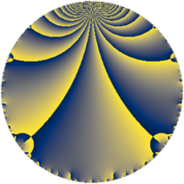# Properties

 Label 169.4.eLevel $169$ Weight $4$ Character orbit 169.e Rep. character $\chi_{169}(23,\cdot)$ Character field $\Q(\zeta_{6})$ Dimension $68$ Newform subspaces $8$ Sturm bound $60$ Trace bound $4$

# Related objects

## Defining parameters

 Level: $$N$$ $$=$$ $$169 = 13^{2}$$ Weight: $$k$$ $$=$$ $$4$$ Character orbit: $$[\chi]$$ $$=$$ 169.e (of order $$6$$ and degree $$2$$) Character conductor: $$\operatorname{cond}(\chi)$$ $$=$$ $$13$$ Character field: $$\Q(\zeta_{6})$$ Newform subspaces: $$8$$ Sturm bound: $$60$$ Trace bound: $$4$$ Distinguishing $$T_p$$: $$2$$

## Dimensions

The following table gives the dimensions of various subspaces of $$M_{4}(169, [\chi])$$.

Total New Old
Modular forms 104 88 16
Cusp forms 76 68 8
Eisenstein series 28 20 8

## Trace form

 $$68 q + 3 q^{2} - 5 q^{3} + 121 q^{4} + 48 q^{6} - 15 q^{7} - 217 q^{9} + O(q^{10})$$ $$68 q + 3 q^{2} - 5 q^{3} + 121 q^{4} + 48 q^{6} - 15 q^{7} - 217 q^{9} - 87 q^{10} + 15 q^{11} - 52 q^{12} + 140 q^{14} - 174 q^{15} - 403 q^{16} + 142 q^{17} + 351 q^{19} + 111 q^{20} - 238 q^{22} - 65 q^{23} - 246 q^{24} - 646 q^{25} - 170 q^{27} - 276 q^{28} - 272 q^{29} + 354 q^{30} + 603 q^{32} + 321 q^{33} - 370 q^{35} - 337 q^{36} + 318 q^{37} + 116 q^{38} + 510 q^{40} + 210 q^{41} + 536 q^{42} - 121 q^{43} - 597 q^{45} - 576 q^{46} + 972 q^{48} - 15 q^{49} - 768 q^{50} - 342 q^{51} - 1378 q^{53} + 510 q^{54} - 288 q^{55} - 710 q^{56} - 9 q^{58} + 525 q^{59} + 326 q^{61} + 916 q^{62} + 1410 q^{63} + 1594 q^{64} + 360 q^{66} + 1077 q^{67} + 1527 q^{68} - 931 q^{69} - 2841 q^{71} - 1425 q^{72} + 565 q^{74} + 1399 q^{75} - 378 q^{76} + 1494 q^{77} + 232 q^{79} - 1923 q^{80} + 1138 q^{81} - 1371 q^{82} - 852 q^{84} - 297 q^{85} + 1033 q^{87} - 816 q^{88} + 1161 q^{89} + 5414 q^{90} + 2812 q^{92} + 1422 q^{93} + 2474 q^{94} + 670 q^{95} - 2487 q^{97} + 1437 q^{98} + O(q^{100})$$

## Decomposition of $$S_{4}^{\mathrm{new}}(169, [\chi])$$ into newform subspaces

Label Dim $A$ Field CM Traces $q$-expansion
$a_{2}$ $a_{3}$ $a_{5}$ $a_{7}$
169.4.e.a $2$ $9.971$ $$\Q(\sqrt{-3})$$ None $$-3$$ $$-2$$ $$0$$ $$24$$ $$q+(-1-\zeta_{6})q^{2}+(-2+2\zeta_{6})q^{3}-5\zeta_{6}q^{4}+\cdots$$
169.4.e.b $2$ $9.971$ $$\Q(\sqrt{-3})$$ None $$6$$ $$7$$ $$0$$ $$-39$$ $$q+(2+2\zeta_{6})q^{2}+(7-7\zeta_{6})q^{3}+4\zeta_{6}q^{4}+\cdots$$
169.4.e.c $4$ $9.971$ $$\Q(\zeta_{12})$$ None $$0$$ $$-4$$ $$0$$ $$0$$ $$q+4\zeta_{12}q^{2}+(-2+2\zeta_{12}^{2})q^{3}+8\zeta_{12}^{2}q^{4}+\cdots$$
169.4.e.d $4$ $9.971$ $$\Q(\zeta_{12})$$ None $$0$$ $$2$$ $$0$$ $$0$$ $$q+\zeta_{12}q^{2}+(1-\zeta_{12}^{2})q^{3}+\zeta_{12}^{2}q^{4}+\cdots$$
169.4.e.e $4$ $9.971$ $$\Q(\zeta_{12})$$ None $$0$$ $$14$$ $$0$$ $$0$$ $$q+5\zeta_{12}q^{2}+(7-7\zeta_{12}^{2})q^{3}+17\zeta_{12}^{2}q^{4}+\cdots$$
169.4.e.f $8$ $9.971$ 8.0.1731891456.1 None $$0$$ $$-10$$ $$0$$ $$0$$ $$q+\beta _{1}q^{2}+(\beta _{2}-3\beta _{7})q^{3}+(-4-3\beta _{2}+\cdots)q^{4}+\cdots$$
169.4.e.g $8$ $9.971$ 8.0.1731891456.1 None $$0$$ $$-10$$ $$0$$ $$0$$ $$q+(-\beta _{1}-2\beta _{3}+\beta _{6}-2\beta _{7})q^{2}+(-1+\cdots)q^{3}+\cdots$$
169.4.e.h $36$ $9.971$ None $$0$$ $$-2$$ $$0$$ $$0$$

## Decomposition of $$S_{4}^{\mathrm{old}}(169, [\chi])$$ into lower level spaces

$$S_{4}^{\mathrm{old}}(169, [\chi]) \cong$$ $$S_{4}^{\mathrm{new}}(13, [\chi])$$$$^{\oplus 2}$$